## How many times it takes a certain number of balls to get a “line” or a “house”, for a given number of players

### Graph of how many times it takes a certain number of balls to get a “line” or a “house”, for a given number of playersGraph of how many times it takes a certain number of balls to get a 'line' or a 'house', for a given number of players

### Introduction

The number of balls to get a “line” may vary more than for houses because you need to get five balls in a row.

The shapes of these graphs are not quite the bell-shaped normal distributions you might expect for random events.

A similar skew was found by a simple simulation of American-style bingo.

It might be that the random-number generator is biased but the same pattern is seen on both Linux and Windows systems which probably use different ways of generating random numbers. Linux may partly use the noise from mouse movements and key-presses whereas Windows may just use a mathematical series.

The numbers on a board in the “British Bingo” game are chosen so that:

• the numbers are in a given range for each column. For example, the first column has numbers in the range 1 to 9,
• each column probably has at least one number.

Therefore not all random selections of numbers from 1 to 90 can be represented on a board in British Bingo. For example: 1, 2, ... 15 cannot. Therefore it may take longer to get a “line” or “house”. This layout of numbers comes from the set of bingo cards I played with at on old folk's home.

The normal distributions below are based on the average and variance of the collected data. There is a discussion of the mathematics elsewhere

### Graph of how many times it takes a certain number of balls to get a “line” or a “house”, for a given number of players, showing approximate normal distributions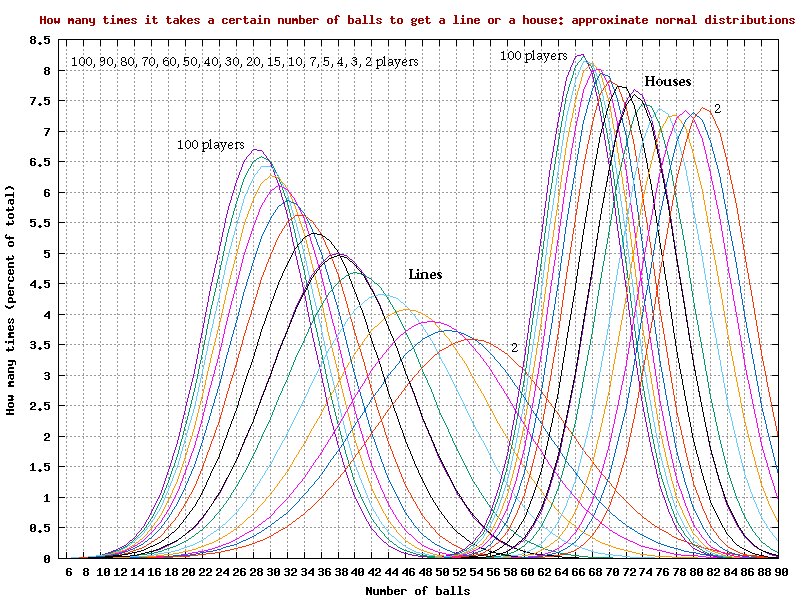Graph of how many times it takes a certain number of balls to get a 'line' or a 'house', for a given number of players, showing approximate normal distributions

### Graphs of how many times it takes a certain number of balls to get a “line”, for a given number of players, in 3D

The scale on the players/depth/z axis on the above 4 graphs is 2, 3, 4, 5, 7, 10, 15, 20, 30, 40, 50, 70, 80, 90, 100, equally spaced

There is a CSV file with the data for the above 4 Excel graphs formatted for the Excel spreadsheet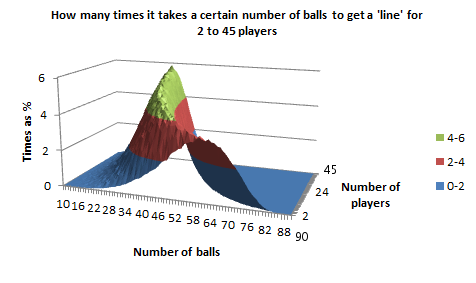Graph of how many times it takes a certain number of balls to get a 'line', for 2 to 35 players, in 3D, as a surface chart in Excel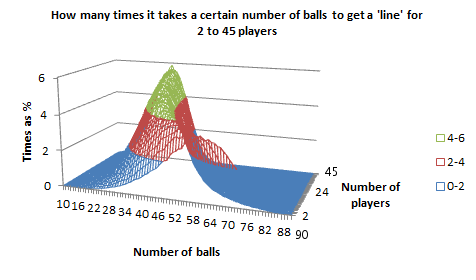Graph of how many times it takes a certain number of balls to get a 'line', for 2 to 35 players, in 3D, as a wireframe chart in Excel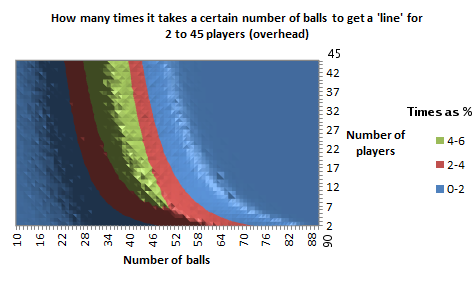Graph of how many times it takes a certain number of balls to get a 'line', for 2 to 35 players, in 3D, as an overhead contour chart in Excel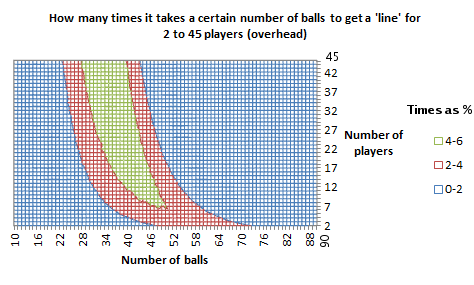Graph of how many times it takes a certain number of balls to get a 'line', for 2 to 35 players, in 3D, as an overhead wireframe chart in Excel

There is a CSV file with the data for the above 4 Excel graphs formatted for the Excel spreadsheet

### Graphs of how many times it takes a certain number of balls to get a “house”, for a given number of players, in 3D

The scale on the players/depth/z axis on the above 4 graphs is 2, 3, 4, 5, 7, 10, 15, 20, 30, 40, 50, 70, 80, 90, 100, equally spaced

There is a CSV file with the data for the above 4 Excel graphs formatted for the Excel spreadsheetGraph of how many times it takes a certain number of balls to get a 'house', for 2 to 35 players, in 3D, as a surface chart in Excel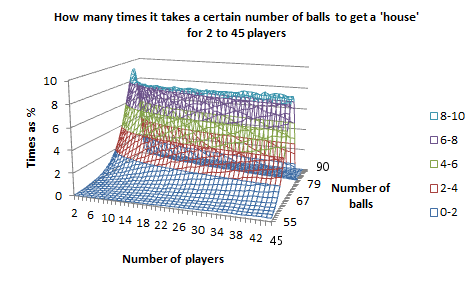Graph of how many times it takes a certain number of balls to get a 'house', for 2 to 35 players, in 3D, as a wireframe chart in Excel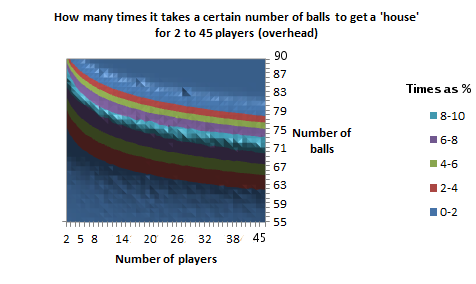Graph of how many times it takes a certain number of balls to get a 'house', for 2 to 35 players, in 3D, as an overhead contour chart in Excel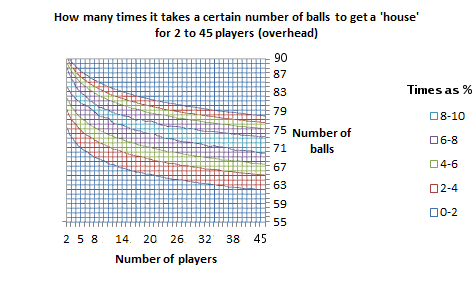Graph of how many times it takes a certain number of balls to get a 'house', for 2 to 35 players, in 3D, as an overhead wireframe chart in Excel

There is a CSV file with the data for the above 4 Excel graphs formatted for the Excel spreadsheet

### Graph of how many times it takes a certain number of balls to get a “line” or a “house”, for 2 playersGraph of how many times it takes a certain number of balls to get a 'line' or a 'house', for 2 players

There are CSV files with the data for the “lines” and “houses”

### Graph of how many times it takes a certain number of balls to get a “line” or a “house”, for 3 players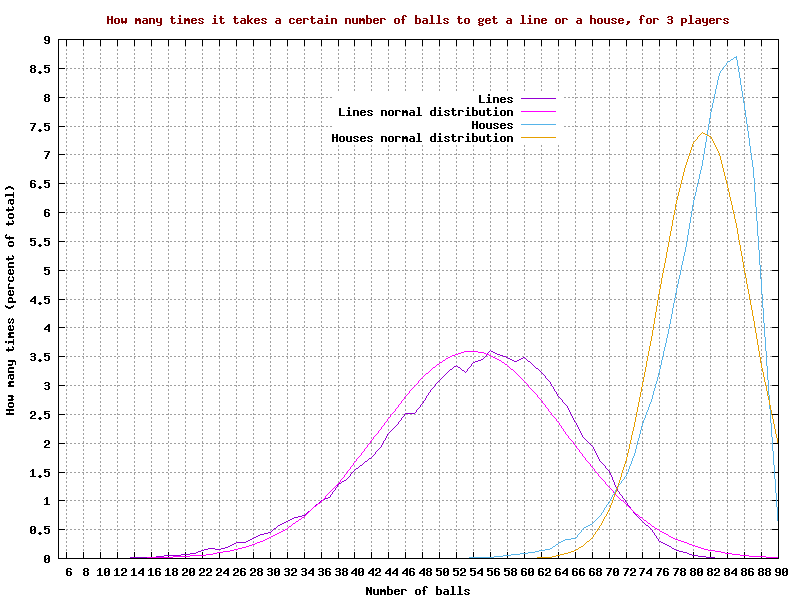Graph of how many times it takes a certain number of balls to get a 'line' or a 'house', for 3 players

There are CSV files with the data for the “lines” and “houses”

### Graph of how many times it takes a certain number of balls to get a “line” or a “house”, for 4 players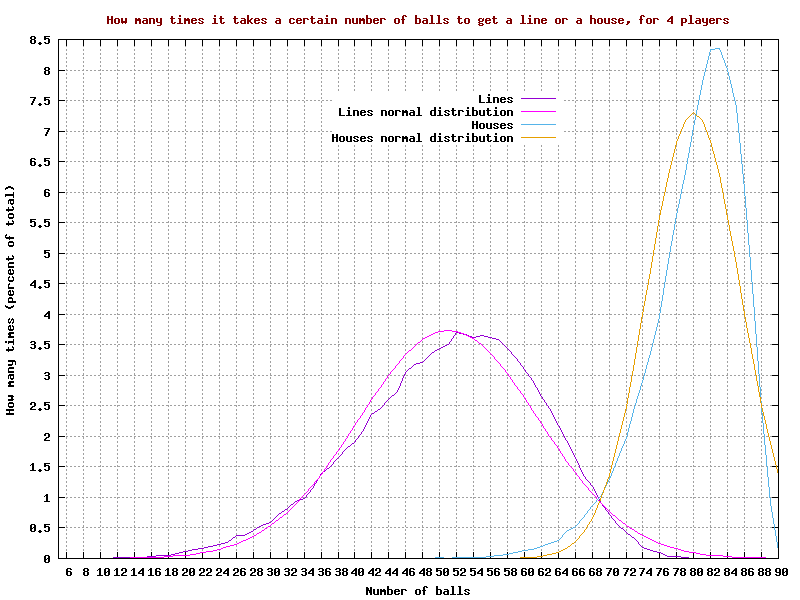Graph of how many times it takes a certain number of balls to get a 'line' or a 'house', for 4 players

There are CSV files with the data for the “lines” and “houses”

### Graph of how many times it takes a certain number of balls to get a “line” or a “house”, for 5 playersGraph of how many times it takes a certain number of balls to get a 'line' or a 'house', for 5 players

There are CSV files with the data for the “lines” and “houses”

### Graph of how many times it takes a certain number of balls to get a “line” or a “house”, for 7 playersGraph of how many times it takes a certain number of balls to get a 'line' or a 'house', for 7 players

There are CSV files with the data for the “lines” and “houses”

### Graph of how many times it takes a certain number of balls to get a “line” or a “house”, for 10 playersGraph of how many times it takes a certain number of balls to get a 'line' or a 'house', for 10 players

There are CSV files with the data for the “lines” and “houses”

### Graph of how many times it takes a certain number of balls to get a “line” or a “house”, for 15 players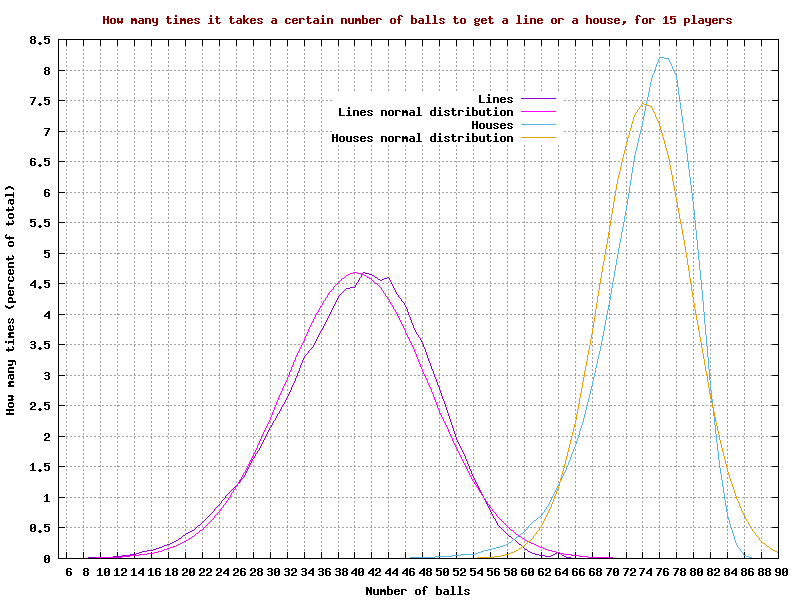Graph of how many times it takes a certain number of balls to get a 'line' or a 'house', for 15 players

There are CSV files with the data for the “lines” and “houses”

### Graph of how many times it takes a certain number of balls to get a “line” or a “house”, for 20 playersGraph of how many times it takes a certain number of balls to get a 'line' or a 'house', for 20 players

There are CSV files with the data for the “lines” and “houses”

### Graph of how many times it takes a certain number of balls to get a “line” or a “house”, for 25 playersGraph of how many times it takes a certain number of balls to get a 'line' or a 'house', for 25 players

There are CSV files with the data for the “lines” and “houses”

### Graph of how many times it takes a certain number of balls to get a “line” or a “house”, for 30 players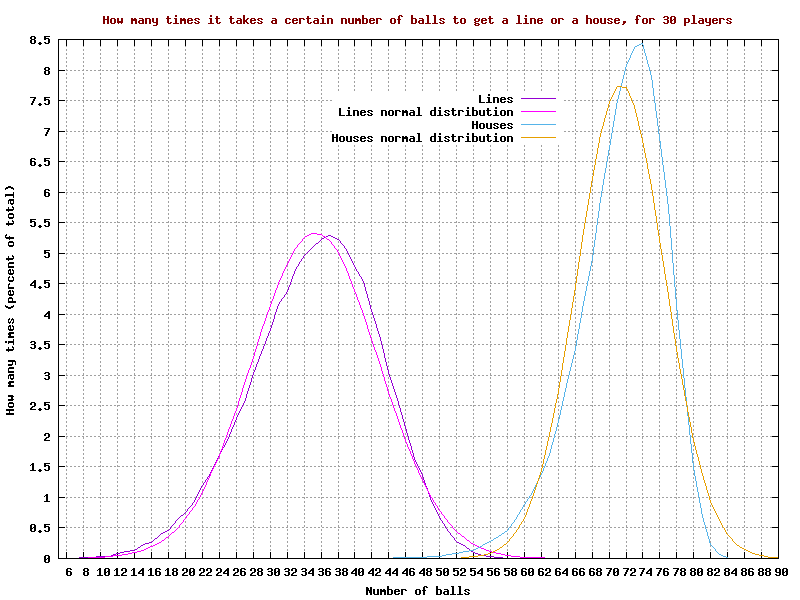Graph of how many times it takes a certain number of balls to get a 'line' or a 'house', for 30 players

There are CSV files with the data for the “lines” and “houses”

### Graph of how many times it takes a certain number of balls to get a “line” or a “house”, for 40 players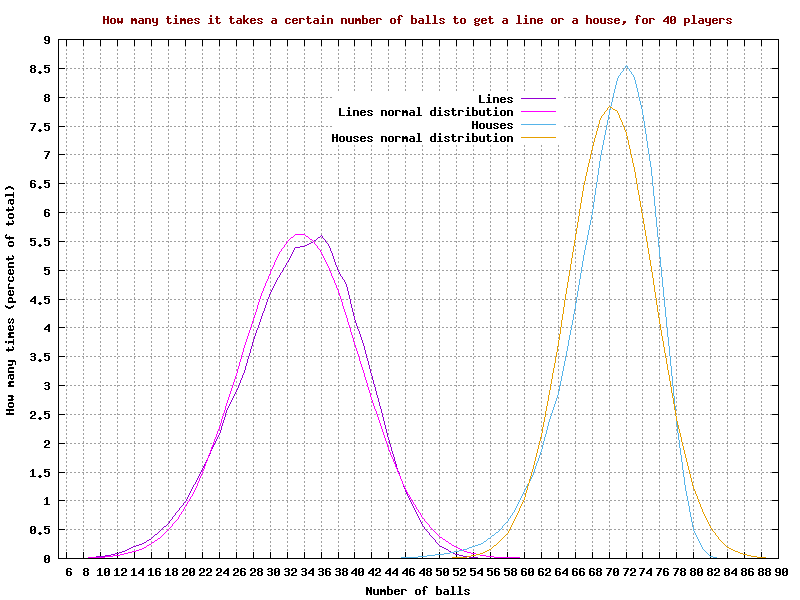Graph of how many times it takes a certain number of balls to get a 'line' or a 'house', for 40 players

There are CSV files with the data for the “lines” and “houses”

### Graph of how many times it takes a certain number of balls to get a “line” or a “house”, for 50 players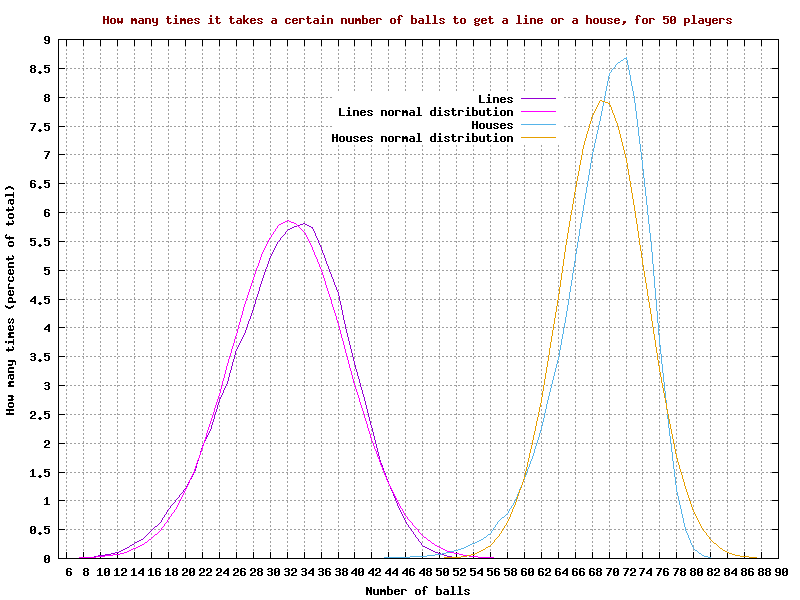Graph of how many times it takes a certain number of balls to get a 'line' or a 'house', for 50 players

There are CSV files with the data for the “lines” and “houses”

### Graph of how many times it takes a certain number of balls to get a “line” or a “house”, for 60 playersGraph of how many times it takes a certain number of balls to get a 'line' or a 'house', for 60 players

There are CSV files with the data for the “lines” and “houses”

### Graph of how many times it takes a certain number of balls to get a “line” or a “house”, for 70 players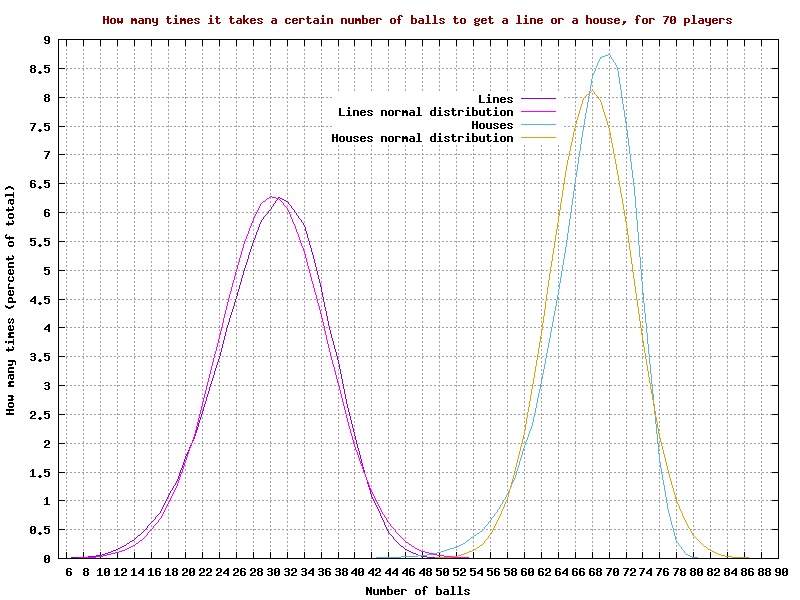Graph of how many times it takes a certain number of balls to get a 'line' or a 'house', for 70 players

There are CSV files with the data for the “lines” and “houses”

### Graph of how many times it takes a certain number of balls to get a “line” or a “house”, for 80 players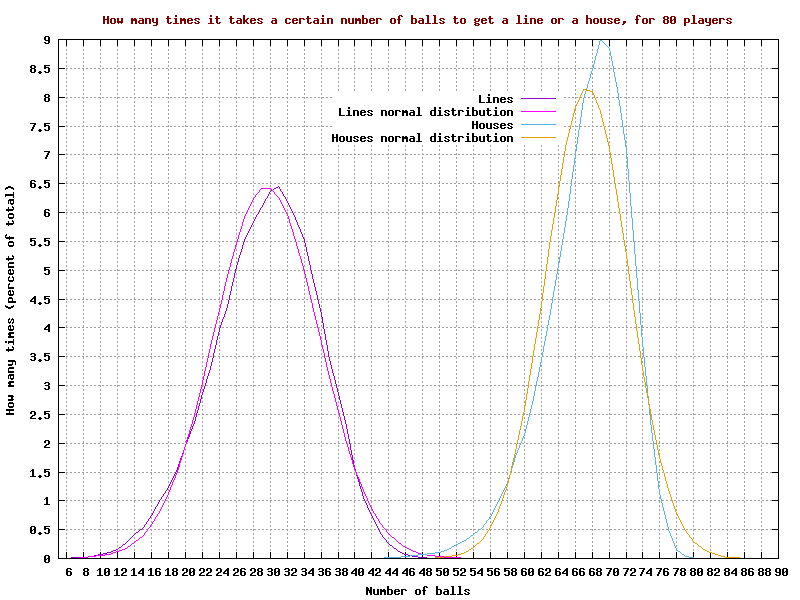Graph of how many times it takes a certain number of balls to get a 'line' or a 'house', for 80 players

There are CSV files with the data for the “lines” and “houses”

### Graph of how many times it takes a certain number of balls to get a “line” or a “house”, for 90 players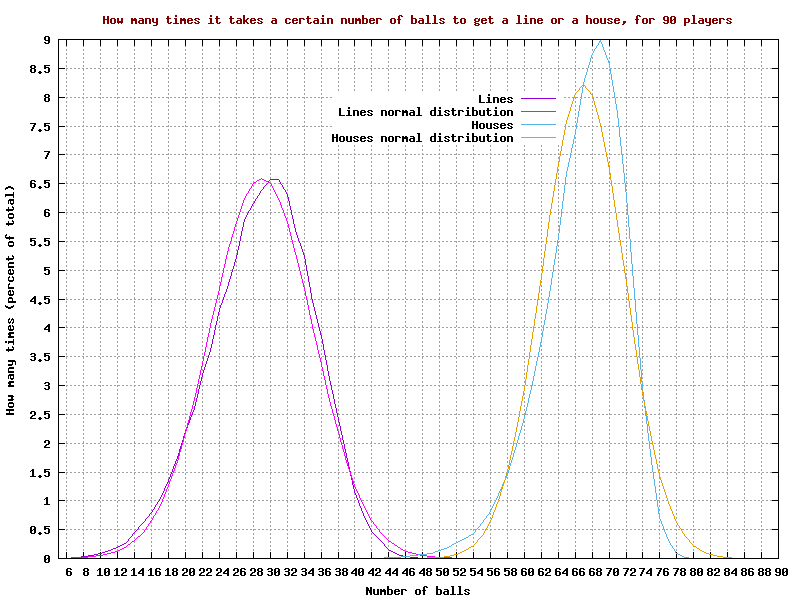Graph of how many times it takes a certain number of balls to get a 'line' or a 'house', for 90 players

There are CSV files with the data for the “lines” and “houses”

### Graph of how many times it takes a certain number of balls to get a “line” or a “house”, for 100 playersGraph of how many times it takes a certain number of balls to get a 'line' or a 'house', for 100 players

There are CSV files with the data for the “lines” and “houses”

There is a file with the raw data for the above graphs together with the scripts used to total that data

## Average number of balls to get a “line” and “house” for a range of number of players

### Graph of the average number of balls to get a “line” for 2 to 50 playersGraph of the average number of balls to get a 'line' for 2 to 50 players

### Graph of the average number of balls to get a “house” for 2 to 50 players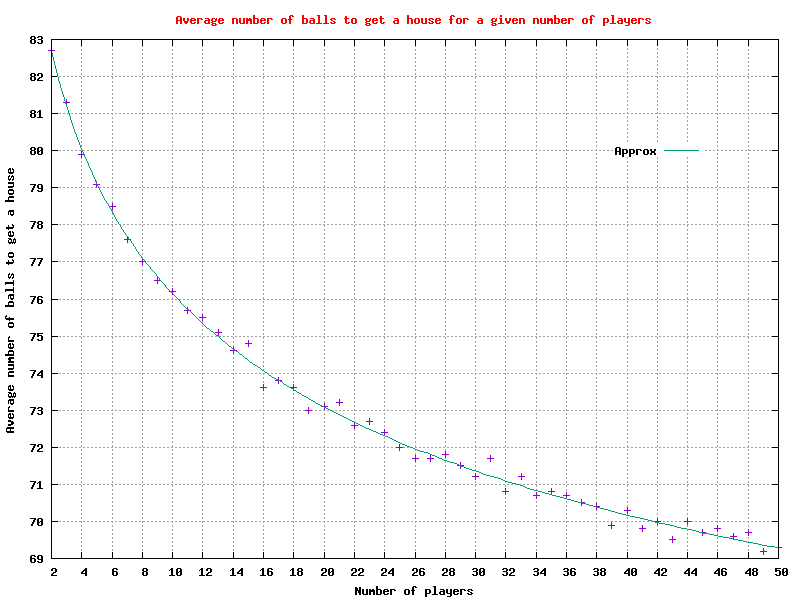Graph of the average number of balls to get a 'house' for 2 to 50 players

### Graph of the average number of balls to get a “line” for 2 to 200 playersGraph of the average number of balls to get a 'line' for 2 to 200 players

### Graph of the average number of balls to get a “house” for 2 to 200 players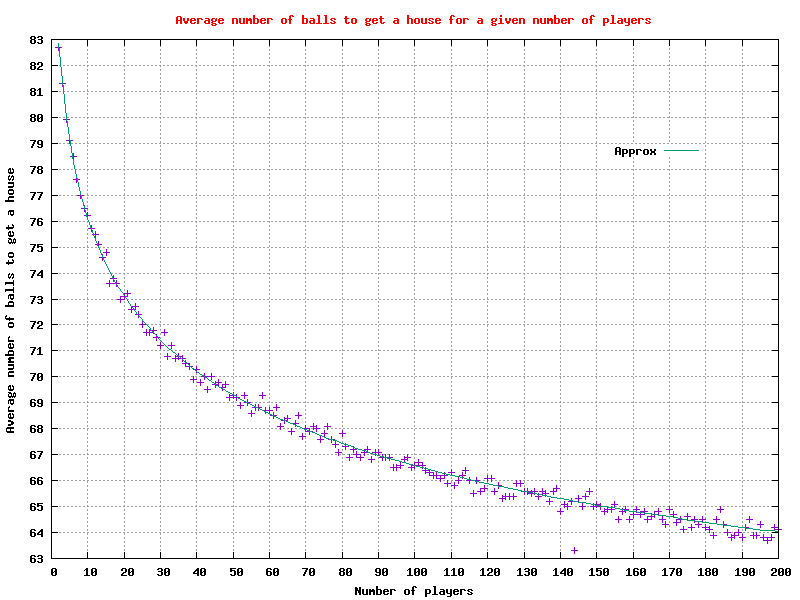Graph of the average number of balls to get a 'house' for 2 to 200 players

### Graph of the average number of balls to get a “line” for 2 to 300,000 players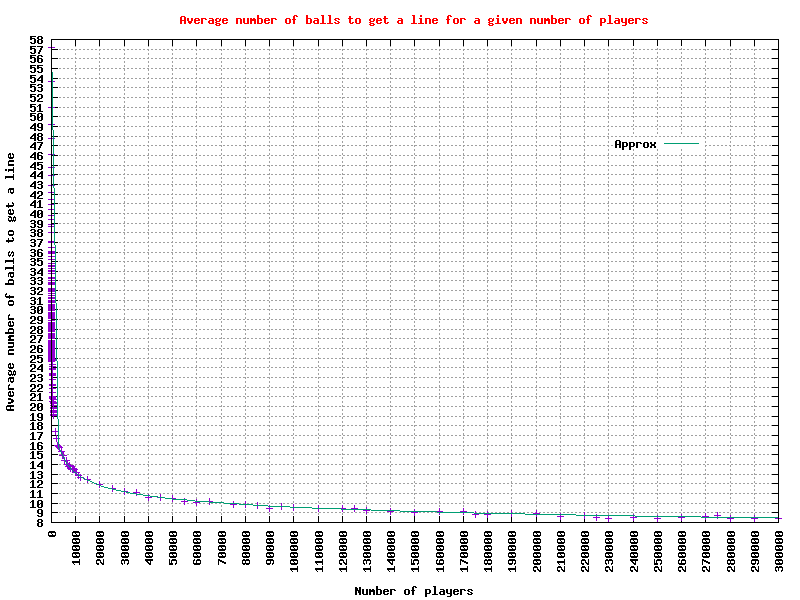Graph of the average number of balls to get a 'line' for 2 to 300,000 players

### Graph of the average number of balls to get a “house” for 2 to 300,000 playersGraph of the average number of balls to get a 'house' for 2 to 300,000 players

### Graph of the average number of balls to get a “line” or “house” for 2 to 50 players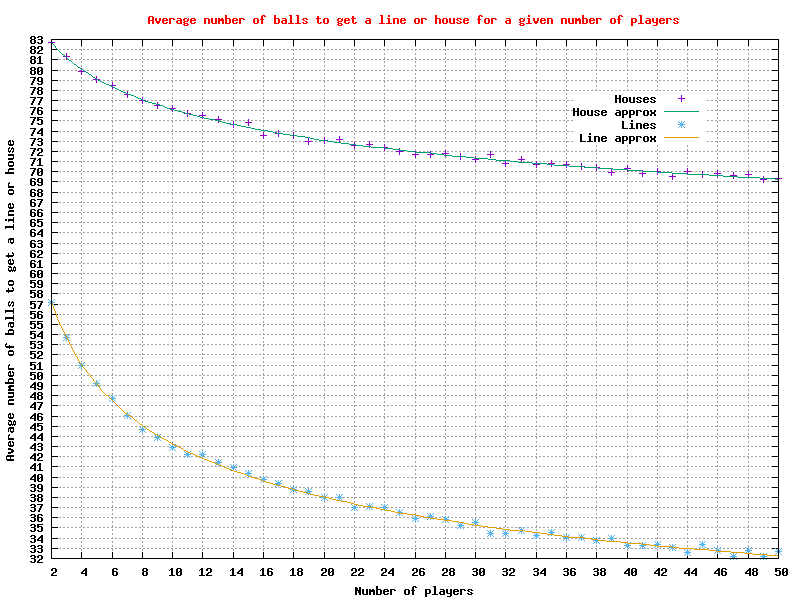Graph of the average number of balls to get a 'line' or 'house' for 2 to 50 players

### Graph of the average number of balls to get a “line” or “house” for 2 to 200 playersGraph of the average number of balls to get a 'line' or 'house' for 2 to 200 players

### Graph of the average number of balls to get a “line” or “house” for 2 to 300,000 players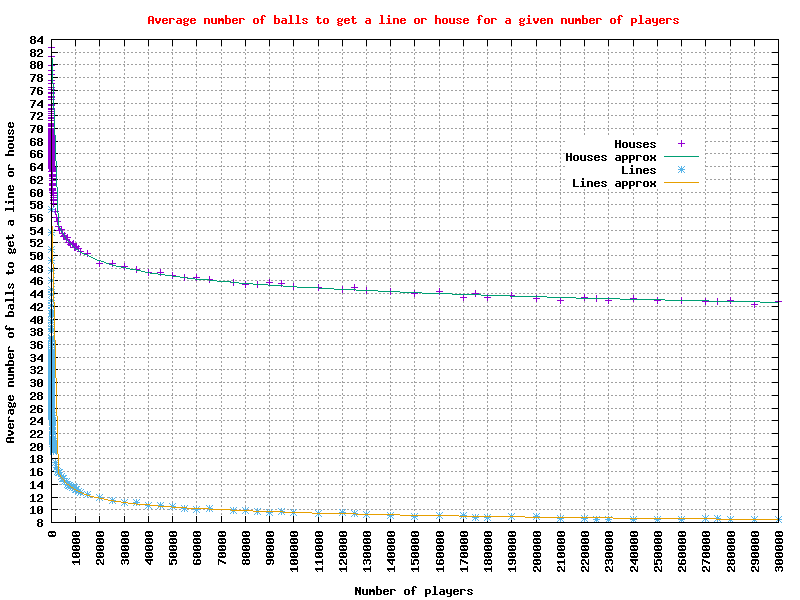Graph of the average number of balls to get a 'line' or 'house' for 2 to 300,000 players

There is a CSV file with the data for the above graphs. We are collecting new data